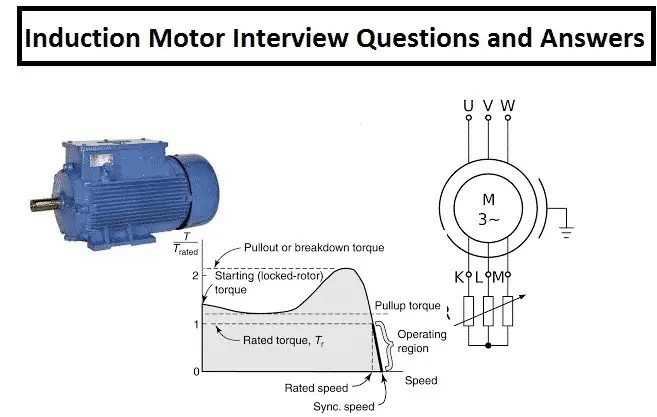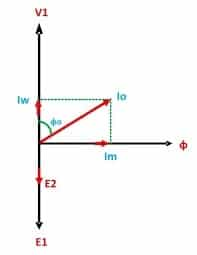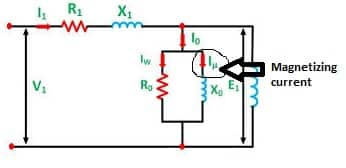# Induction Motor Interview Questions and Answers Part-2

Last Updated on February 11, 2022 by ElectricalvoltInduction Motor Interview Questions and Answers is very important for competitive exams and interview purpose. The below questions and answers are very conceptually explained

## What is the stand still condition of induction motor?

When the motor is at standstill, the relative speed of the rotating magnetic field and the rotor is equal to 1. The difference in the synchronous speed of the motor and the actual speed of the rotor is unity. In other words, the slip of the motor is unity when the rotor is at standstill.

slip(s)= (Ns-Nr)/Ns

At standstill, Speed of the motor Nr=0

s= (Ns-0)/Ns
=Ns/Ns
=1

At start, the slip of the motor is 1. The motor draws maximum current when it starts accelerating from its standstill position. As the motor speed pick-up, the current starts reducing.

## What should be the no. load current of induction motor?The current drawn by the induction motor when it is not coupled to the driven equipment is called no-load current of the motor. The no-load current produces the magnetic field in the motor. There are two parts of no-load current.

1. Magnetizing component of the current
2. Loss components( Hysteresis and eddy current loss)

The no-load current is the vector sum of the magnetizing & no-load loss components current.## How is a rotating magnetic field is produced in a three phase induction motor?

Three Phase Induction Motor works on the principal of rotating magnetic field (R. M. F.). The rotating magnetic field can be defined as the field or flux having constant amplitude but whose axis is continuously rotating in a plane with a certain speed.

In three phase induction motors such a rotating magnetic field is produced by supplying currents to a set of three stationary winding, with the help of three phase a.c. Supply.

The current carrying winding produce the magnetic field or flux.

Aluminium Armoured cable

Select the cable size sqmm equal to the full load current of the motor.

For handling 100 amp current select 95 sqmm cable Al cable.

Always choose higher size cable

1. Reduces the IR drop, low losses
2. Can handle more fault current
3. System reliability is better

Connect inductance meter between the two coils of the star connected coils. Divide the measued value by 2 to get inductance of the coil.

Or- In absence of  inductance meter, the inductance of the coil can be measured by the following method.

1. Measure the resistance(R) of coils(phase to phase)
2. Feed AC voltage (V), 50 Hz to coils and measure the current(I)
3. Calculate impedance of the coils Z=V/I
4. Determine reactance(XL) of the coils by XL=√(Z^2-R^2)
5. Calculate Inductance of the coils by L= XL/2*π*f
6. Divide the value of L by 2 is the inductance of the coil.

The magnetizing current is essential for setting up a magnetic field in the air gap of the motor.

Without magnetizing current, the magnetic field can’t be generated, and the motor operation is not possible. The stator winding is inductive in nature and when applied AC to the stator, it draws current that lags the applied voltage.There are two types of induction motor.

1. Squirrel Cage Induction Motor(SCIM)

The rotor conductors of the SCIM are short-circuited. The motor is rugged as the connection of the rotor is not taken outside for external connections. However, the starting torque of the motor is low.

2. Slip ring Induction Motor(SRIM)

The connection of the rotor is taken out through the slip ring and carbon brushes and connected to the external resistances. The starting current of the motor is low, and a starting torque of the motor is better than the SCIM.

The RPM data is engraved into the nameplate of the motor. The synchronous speed of the motor is 3000,1500,1000,750,600 RPM etc for 2,4,6,8,10 poles induction motor( Ns=120f/P)… The actual speed of the motor is somewhat less than the synchronous speed of the motor. The actual speed of the 2 poles machine may be in the range of 2900 RPM to 2980 RPM.

If the nameplate of the motor is missing, connect three phase supply to the motor and measure the actual speed of the motor with a tachometer. For example, if the measured speed of the motor is 720 RPM, it means it is an eight poles machine.

The slip ring motor has high starting torque as the resistance of the rotor winding is increased by adding the external resistance to it. The starting torque is increased as the power factor of the rotor circuit gets improved during starting.

T= K* Flux* Rotor Current* Power Factor

Power factor = R/Z

=(Rotor winding Resistance+External Resistance)/Z

However, it is not possible to add an external resistance to the rotor of squirrel cage induction motor.The starting torque of squirrel cage induction motor can be improved by use of double cage rotor.

When the motor is started the current flows through the upper cage winding which has more resistance. When the motor attains its base speed the most of the current flows through the lower cage of the motor.

The rotor of the slip ring induction motor is connected to the external resistances. When the motor is started the total external resistance get added in the rotor winding resistance and the value of the resistances get gradually decreased with the acceleration of the motor and becomes zero when the motor attains its full rated speed. The purpose of adding external resistance to the rotor is to increase the starting torque and to limit the starting current of the motor.

If the slip rings are shorted, a huge current will flow in the stator and the breaker will trip with the over-current fault. If the breaker does not trip the electrical power network will experience heavy stress and the motor is likely to get damaged in this situation.

The flash-over in the slip ring is a typical problem that occurs with slip ring motors. The slip ring must be cleaned periodically by blowing the carbon particles in the slip ring chamber. At the time of starting, the maximum rotor voltage is produced at the slip rings and any carbon or dust particles, moisture at the slip rings may cause insulation breakdown.

If slip ring motor is to be operated in the polluted environment, the slip ring must be cleaned periodically or keep the creepage distance more than the designed value.

The synchronous speed of the 6 poles induction motor is,

Ns=120f/P= 120*50/6= 1000 RPM

The full load speed of the rotor=1140 RPM

The full load speed can’t be more than the synchronous speed of the motor if the motor is working in the motoring zone.

The rotor speed can be more than the synchronous speed of the motor if the rotor is rotated with an external power source.

The slip = (Ns-Nr)/Ns

=(1000–1140)/1000

=-140/1000

=- 0.14

= – 14 %

The motor will act as a generator in this case, and the active energy starts feeding in the mains supply source.

Related PostsSatyadeo Vyas, M.Tech,M.B.A. is an electrical engineer and has more than 36 years of industrial experience in the operation, maintenance, and commissioning of electrical and instrumentation projects. He has good knowledge of electrical, electronics, and instrumentation engineering.

1.